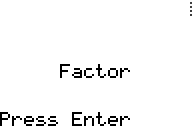FactorTitle screen of Factor.
Original author(s) Gregory McLeod
Developer(s) Gregory McLeod
Publisher(s) McLeodGaming
Release date 2003
Type Mathematics
Platform(s) TI-83 Plus
Media TI-BASIC Program

Factor is a numerical analysis tool made in 2003 for the TI-83 Plus calculator by Gregory McLeod and published by McLeodGaming. It is made to automatically calculate the factors of a number entered.

## Function

To use the tool, the user must enter the number they desire to know the factors of on the screen asking for this. The number cannot be less than or equal to 1, as the program is unable to factor it. After confirming with Enter, the program will then slowly determine each number that is a factor of the number selected by calculating this with each whole number from 1 to half of the number, at which point the only remaining factor is the number itself. Each factor that the program finds is displayed on the screen as it factors, and when complete, the user is able to view and scroll through these factors.

## Trivia

• Due to memory limitations, Factor is unable to find the factors of numbers greater than 357, in which case it will crash while factoring.